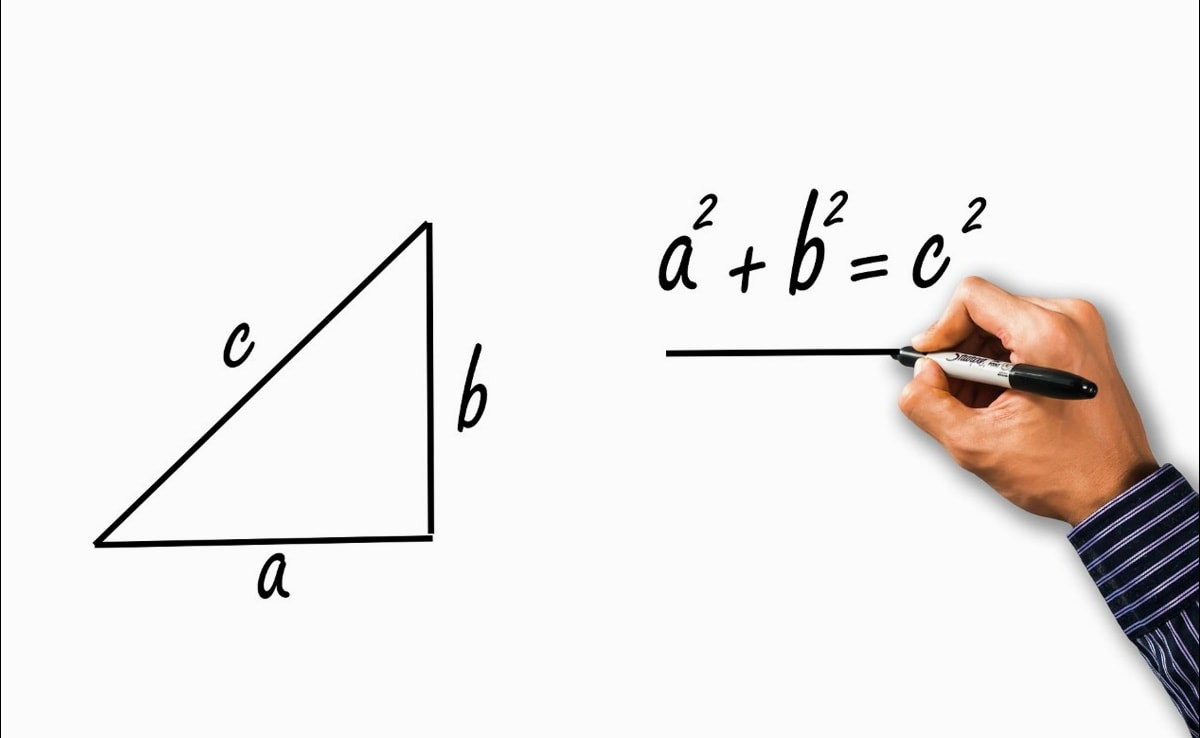# Budapest Post

Cum Deo pro Patria et Libertate
Budapest, Europe and world news# US Teens Discover New Proof For The Pythagoras' Theorem, Stun Mathematicians

The students said they can prove the theorem by using trigonometry and without circular reasoning.
Two American high school students have stunned mathematicians after they claimed that they discovered a new way to prove Pythagoras' theorem by using trigonometry- a feat mathematicians thought was impossible, Guardianreported.

Calcea Johnson and Ne'Kiya Jackson, who are seniors at St. Mary's Academy in New Orleans, presented their findings on March 18 at the American Mathematical Society's (AMS) Spring Southeastern Sectional Meeting.

''Their groundbreaking lecture from the research is historic. High School students are generally not presenters at the American Mathematical Society Meeting,'' the school's announcement notes.

Notably, the 2,000-year-old Pythagorean theorem states that the sum of the squares of a right triangle's two shorter sides is the same as the square of the hypotenuse, the third side opposite the right angle. Students around the world learned the notation expressing the theory as a2+b2=c2. However, mathematicians have struggled to find a definitive proof for the theorem which would not only show that it works but explain why it does.

''In the 2000 years since trigonometry was discovered, it's always been assumed that any alleged proof of Pythagoras's Theorem based on trigonometry must be circular,'' they told an audience at the American Mathematical Society Southeastern Regional Conference.

''In fact, in the book containing the largest known collection of proofs (The Pythagorean Proposition by Elisha Loomis) the author flatly states that ‘There are no trigonometric proofs because all the fundamental formulae of trigonometry are themselves based upon the truth of the Pythagorean Theorem.''

The students further said they can prove the theorem by using trigonometry and without circular reasoning.

But "that isn't quite true," the teenagers wrote in the abstract. "We present a new proof of Pythagoras's Theorem which is based on a fundamental result in trigonometry — the Law of Sines — and we show that the proof is independent of the Pythagorean trig identity sin2x+cos2x=1."

However, the findings have not yet been accepted into a peer-reviewed journal. According to Live Science, it's still too soon to say whether their proof will ultimately hold up.